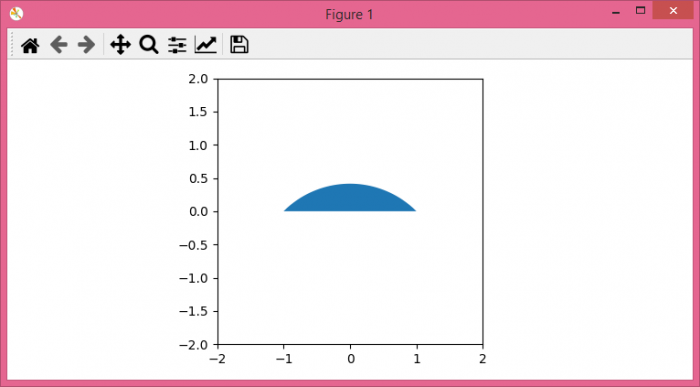# How to draw a filled arc in Matplotlib?

To draw a filled arc in matplotlib, we can take the following steps −

• Set the figure size and adjust the padding between and around the subplots.

• Create a figure and a set of subplots.

• Initialize two variables, r, yoff.

• Create x and y data points using Numpy.

• Fill the area between x and y plots.

• Set the axis aspect and draw the figure canvas.

• To display the figure, use show() method.

## Example

import matplotlib.pyplot as plt
import numpy as np

plt.rcParams["figure.figsize"] = [7.50, 3.50]
plt.rcParams["figure.autolayout"] = True

fg, ax = plt.subplots(1, 1)

r = 2.
yoff = -1

x = np.arange(-1., 1.05, 0.05)
y = np.sqrt(r - x ** 2) + yoff

ax.fill_between(x, y, 0)
ax.axis([-2, 2, -2, 2])
ax.set_aspect("equal")

fg.canvas.draw()

plt.show()

## Output